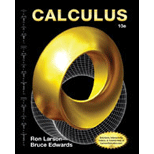# The value of c in the interval ( a , b ) such that f ′ ( c ) g ′ ( c ) = f ( b ) − f ( a ) g ( b ) − g ( a ) by applying extended mean value theorem to functions f ( x ) = x 3 and g ( x ) = x 2 + 1 in the interval [ 0 , 1 ] .### Calculus

10th Edition
Ron Larson + 1 other
Publisher: Cengage Learning
ISBN: 9781285057095### Calculus

10th Edition
Ron Larson + 1 other
Publisher: Cengage Learning
ISBN: 9781285057095

#### Solutions

Chapter 8.7, Problem 91E
To determine

## Expert Solution

### Want to see the full answer?

Check out a sample textbook solution.

### Want to see this answer and more?

Experts are waiting 24/7 to provide step-by-step solutions in as fast as 30 minutes!*

*Response times may vary by subject and question complexity. Median response time is 34 minutes for paid subscribers and may be longer for promotional offers.